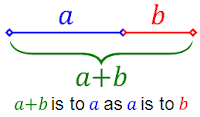# Fibonacci Series and Golden ratio

Golden Ratio:If the ratio of a+b to a is equal to a to b then that ratio is called Golden ratio

$\displaystyle\frac{{a + b}}{a} = \displaystyle\frac{a}{b}$ = $\varphi$

This greek letter "$\varphi$" is called golden ratio which is equal to $\displaystyle\frac{{1 + \sqrt 5 }}{2}$
From the above ratio, we get
$\displaystyle\frac{{a + b}}{a} = 1 + \frac{b}{a} = \varphi \Rightarrow 1 + \frac{1}{\varphi } = \varphi$
$\Rightarrow \varphi ^2 - \varphi - 1 = 0$
The roots of the above equation are $\displaystyle\frac{{1 \pm \sqrt 5 }}{2}$ But we have to take only the positive value $\displaystyle\frac{{1 + \sqrt 5 }}{2}$ as this ratio is positive.
In general $\displaystyle\frac{{1 + \sqrt 5 }}{2}$ is represented with $\varphi$ and $\displaystyle\frac{{1 - \sqrt 5 }}{2}$ is represented with ${\hat \varphi }$
Here $\varphi$ = 1.618 and ${\hat \varphi }$ = - 0.618

First 20 Fibonnaci numbers : 1, 1, 2, 3, 5, 8, 13, 21, 34, 55, 89, 144, 233, 377, 610, 987, 1597, 2584, 4181, 6765

Finding nth term of a Fibonacci series:

nth term of a fibonnacci series 1, 1, 2, 3, 5, 8, ........... = $\displaystyle\frac{{\left( {\displaystyle\frac{{1 + \sqrt 5 }}{2}} \right)^n - \left( {\displaystyle\frac{{1 - \sqrt 5 }}{2}} \right)^n }}{{\sqrt 5 }}$

Copy paste this in google chrome bar ((1+sqrt 5)/2)^n/sqrt 5-((1-sqrt 5)/2)^n/sqrt 5 Change n by your required number.

For quick Approximation you can use this (1.618^n)+(-0.618)^n) / sqrt 5

Sum of the fibobbacci series ${F_1} + {F_2} + {F_3} + ...... + {F_n} = {F_{n + 2}} - 1$

Some Amazing results:

1. Sum of the squares of n fibonacci terms is equal to the product of nth term and (n+1)th term.
For example, $1^2 + 1^2 + 2^2 + 3^2 + 5^2 + 8^2 + 13^2 + 21^2 + 34^2 + 55^2 =$55 x 89 = 4895

2. Difference between any two consecutive Fibonacci squares is equal to another Fibonacci number
$F_n ^2 - F_{n - 2} ^2 = F_{2n - 2}$
$F_6 ^2 - F_4 ^2 = 8^2 - 3^2 = 55 = F_{10}$
$F_7 ^2 - F_5 ^2 = 13^2 - 5^2 = 144 = F_{12}$

3. The product of two consecutive fibonnacci numbers is equal to the square of the middle number $\pm$ 1
$F_n \times F_{n - 2} = F_n ^2 \pm 1$
$F_4 \times F_6 = 3 \times 8 = 24 = 5^2 - 1 = F_5 ^2 \pm 1$

Fibonacci series starting with 1 and 6:

If the Fibonacci series start with 1 and 6, then the series goes like this. 1, 6, 7, 13, 20, 33, 53, 88, ...
In this case how do we find the general term of this series.
Here $x_0 = 1$ and  $x_1 = 6$
So It takes the form of $x_n = A\varphi ^n + B\hat \varphi ^n$
For x = 0, 1 = A + B --------(1)
For x = 1, $6 = A\varphi + B\hat \varphi$ -------(2)
Here $\varphi = \displaystyle\frac{{1 + \sqrt 5 }}{2}$ and $\hat \varphi = \displaystyle\frac{{1 - \sqrt 5 }}{2}$
Multiplying the (1) with $\varphi$ and Substracting from 2,
$A\varphi + B\hat \varphi = 6$
$A\varphi + B\varphi = \varphi$
---------------------------
$B(\hat \varphi - \varphi ) = 6 - \varphi$
$B = \displaystyle\frac{{6 - \varphi }}{{\hat \varphi - \varphi }} = \frac{{\varphi - 6}}{{\sqrt 5 }} = \frac{{\frac{{\sqrt 5 + 1}}{2} - 6}}{{\sqrt 5 }} = \frac{{\sqrt 5 - 11}}{{2\sqrt 5 }} = \frac{{5 - 11\sqrt 5 }}{{10}}$
A = 1- B = $\displaystyle\frac{{5 + 11\sqrt 5 }}{{10}}$

Now general term of this series = $\left( {\displaystyle\frac{{5 + 11\sqrt 5 }}{{10}}} \right)\left( {\displaystyle\frac{{1 + \sqrt 5 }}{2}} \right)^n + \left( {\displaystyle\frac{{5 - 11\sqrt 5 }}{{10}}} \right)\left( {\displaystyle\frac{{1 - \sqrt 5 }}{2}} \right)^n$

Sum of the n terms of this series =  ${F_1} + {F_2} + {F_3} + ...... + {F_n} = {F_{n + 2}} - 6$